We do not support landscape mode yet.
Please go back to portrait mode for the best experience
Question of The Day05-10-2019

On a certain amount at the rate of 12% per annum for 1 year and 6 months the compound interest is Rs. 468. What will be the principal amount?

Correct Answer : a ) Rs. 2500

Explanation :

Quick Approach

Given: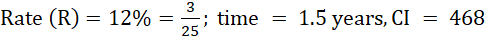Considering the rate, we should assume the principal to be such that it will get completely divided by rate.

So,

Let us assume the principal amount to be 625

Then,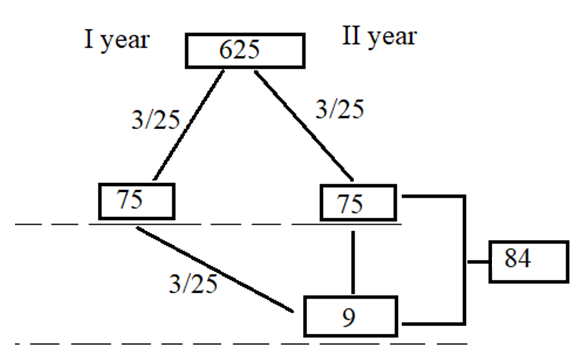So, from the figure we can say for principal interest to be 625, 1st year CI will be 75 and 2nd year CI will be 84.

Thus,

CI for 6 months will be 84/2 =42

Thus, total CI will be 75 + 42 = 117

Now, for the principal of 625, CI will be 117

Then, for CI of 468 principal would be = Rs. 2500

Hence, (a) is the correct answer.

Basic Approach

Given:Compound interest (CI) for 1 year is equal to simple interest (SI) for 1 year.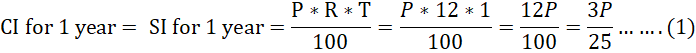Now, CI for 2nd year will be the sum of CI for 1 year and 12% of CI for 1 year

Thus,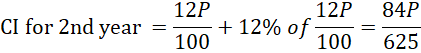As, we have to calculate the SI of 1year and 6 months thus, we will take half of the compound interest for 2nd year

So,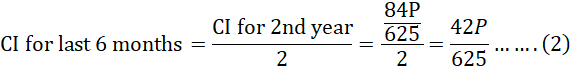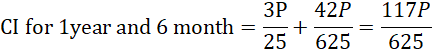According to the question, compound interest for 1 year 6 months is equal to Rs. 438

So,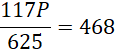P = Rs. 2500

Hence, (a) is the correct answer.

Questions on Simple Interest and Compound Interest are asked in the Quantitative Aptitude section of various government exams like SSC CGL, SSC MTS, SSC CPO, SSC CHSL, IBPS PO, IBPS SO, IBPS CLERK,  RRB JE, RRB NTPC, RRB GROUP D, etc. Try and attempt free mock tests at PendulumEdu and learn easy and quick methods to solve such questions. One can also clear all exam related doubts through PendulumEdu’s one-to-one session.